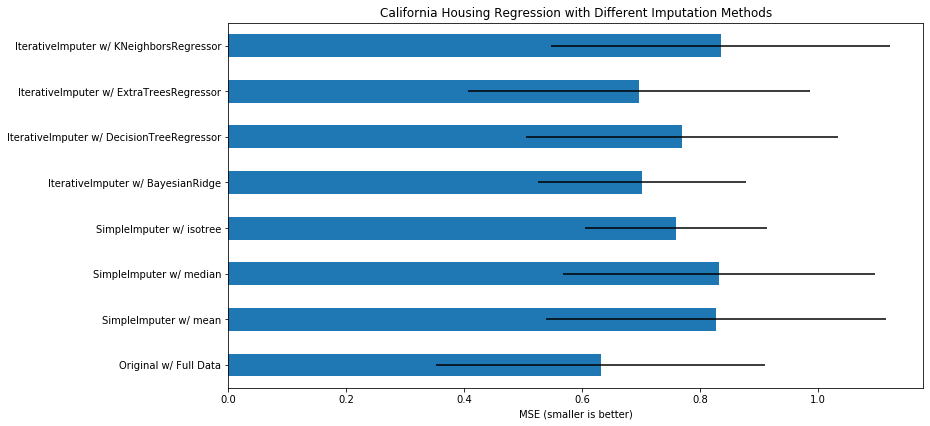# Imputation of missing values with IsoTree¶

This is a short example about imputation of missing values with the package isotree (Isolation Forest and variations), which produces imputations by taking the values from observations in the terminal nodes of each tree in which an observation with missing values falls at prediction time, combining the non-missing values of the other observations as a weighted average according to the depth of the node and the number of observations that fall there. This is not related to how the model handles missing values internally, but is rather meant as a faster way of imputing by similarity. Quality is not as good as chained equations, but the method is a lot faster and more scalable. Recommended to use non-random splits when used as an imputer.

The example here is copy-pasted from SciKit-Learn's usage guide for their imputer, and just adds a few extra lines that do the job with this package.

Original code was taken from this link:

https://scikit-learn.org/stable/auto_examples/impute/plot_iterative_imputer_variants_comparison.html#sphx-glr-auto-examples-impute-plot-iterative-imputer-variants-comparison-py

In :
import numpy as np
import matplotlib.pyplot as plt
import pandas as pd
%matplotlib inline

### As of 2019-11-02, SciKit-Learn's example throws lots of convergence warnings
import warnings
warnings.filterwarnings("ignore")

# To use this experimental feature, we need to explicitly ask for it:
from sklearn.experimental import enable_iterative_imputer  # noqa
from sklearn.datasets import fetch_california_housing
from sklearn.impute import SimpleImputer
from sklearn.impute import IterativeImputer
from sklearn.linear_model import BayesianRidge
from sklearn.tree import DecisionTreeRegressor
from sklearn.ensemble import ExtraTreesRegressor
from sklearn.neighbors import KNeighborsRegressor
from sklearn.pipeline import make_pipeline
from sklearn.model_selection import cross_val_score

N_SPLITS = 5

rng = np.random.RandomState(0)

X_full, y_full = fetch_california_housing(return_X_y=True)
# ~2k samples is enough for the purpose of the example.
# Remove the following two lines for a slower run with different error bars.
X_full = X_full[::10]
y_full = y_full[::10]
n_samples, n_features = X_full.shape

# Estimate the score on the entire dataset, with no missing values
br_estimator = BayesianRidge()
score_full_data = pd.DataFrame(
cross_val_score(
br_estimator, X_full, y_full, scoring='neg_mean_squared_error',
cv=N_SPLITS
),
columns=['Full Data']
)

# Add a single missing value to each row
X_missing = X_full.copy()
y_missing = y_full
missing_samples = np.arange(n_samples)
missing_features = rng.choice(n_features, n_samples, replace=True)
X_missing[missing_samples, missing_features] = np.nan

# Estimate the score after imputation (mean and median strategies)
score_simple_imputer = pd.DataFrame()
for strategy in ('mean', 'median'):
estimator = make_pipeline(
SimpleImputer(missing_values=np.nan, strategy=strategy),
br_estimator
)
score_simple_imputer[strategy] = cross_val_score(
estimator, X_missing, y_missing, scoring='neg_mean_squared_error',
cv=N_SPLITS
)

# This is the piece of code that adds imputations with isotree
from isotree import IsolationForest
estimator = make_pipeline(
IsolationForest(build_imputer=True, min_imp_obs=1, prob_pick_pooled_gain=1, ntry=15),
br_estimator
)
score_simple_imputer["isotree"] = cross_val_score(
estimator, X_missing, y_missing, scoring='neg_mean_squared_error',
cv=N_SPLITS
)
##### END OF NEW ADDITION #########

# Estimate the score after iterative imputation of the missing values
# with different estimators
estimators = [
BayesianRidge(),
DecisionTreeRegressor(max_features='sqrt', random_state=0),
ExtraTreesRegressor(n_estimators=10, random_state=0),
KNeighborsRegressor(n_neighbors=15)
]
score_iterative_imputer = pd.DataFrame()
for impute_estimator in estimators:
estimator = make_pipeline(
IterativeImputer(random_state=0, estimator=impute_estimator),
br_estimator
)
score_iterative_imputer[impute_estimator.__class__.__name__] = \
cross_val_score(
estimator, X_missing, y_missing, scoring='neg_mean_squared_error',
cv=N_SPLITS
)

scores = pd.concat(
[score_full_data, score_simple_imputer, score_iterative_imputer],
keys=['Original', 'SimpleImputer', 'IterativeImputer'], axis=1
)

# plot boston results
fig, ax = plt.subplots(figsize=(13, 6))
means = -scores.mean()
errors = scores.std()
means.plot.barh(xerr=errors, ax=ax)
ax.set_title('California Housing Regression with Different Imputation Methods')
ax.set_xlabel('MSE (smaller is better)')
ax.set_yticks(np.arange(means.shape))
ax.set_yticklabels([" w/ ".join(label) for label in means.index.get_values()])